• 定义相关系数矩阵PHO2. 调用pcacov函数根据相关系数矩阵作主成分分析3. 为了更加直观，以元胞数组形式显示结果4. 以元胞数组形式显示主成分表达式 1. 定义相关系数矩阵PHO PHO = [1 0.79 0.36 0.76 0.25 0.51 ...
文章目录1. 定义相关系数矩阵PHO2. 调用pcacov函数根据相关系数矩阵作主成分分析3. 为了更加直观，以元胞数组形式显示结果4. 以元胞数组形式显示主成分表达式

1. 定义相关系数矩阵PHO
PHO = [1     0.79    0.36    0.76    0.25    0.51
0.79  1       0.31    0.55    0.17    0.35
0.36  0.31    1       0.35    0.64    0.58
0.76  0.55    0.35    1       0.16    0.38
0.25  0.17    0.64    0.16    1       0.63
0.51  0.35    0.58    0.38    0.63    1
];

2. 调用pcacov函数根据相关系数矩阵作主成分分析
% 返回主成分表达式的系数矩阵COEFF，返回相关系数矩阵的特征值向量latent和主成分贡献率向量explained
[COEFF,latent,explained] = pcacov(PHO)3. 为了更加直观，以元胞数组形式显示结果
result1(1,:) = {'特征值', '差值', '贡献率', '累积贡献率'};
result1(2:7,1) = num2cell(latent);
result1(2:6,2) = num2cell(-diff(latent));
result1(2:7,3:4) = num2cell([explained, cumsum(explained)])4. 以元胞数组形式显示主成分表达式
s = {'标准化变量';'x1:身高';'x2:坐高';'x3:胸围';'x4:手臂长';'x5:肋围';'x6:腰围'};
result2(:,1) = s ;
result2(1, 2:4) = {'Prin1', 'Prin2', 'Prin3'};
result2(2:7, 2:4) = num2cell(COEFF(:,1:3))展开全文主成分分析
• 1. 已知信号矩阵sigmat, 协方差矩阵(covariance matrix) 可以用matlab函数cov(sigmat)求得...2. 相关矩阵跟相关系数矩阵是同一个矩阵，相关系数即为相关矩阵的矩阵元，已知协方差矩阵可以求相关矩阵：corrcov(covmat);
转载自： http://blog.csdn.net/myj0513/article/details/24188081

1. 已知信号矩阵sigmat, 协方差矩阵(covariance matrix) 可以用matlab函数cov(sigmat)求得；
2. 相关矩阵跟相关系数矩阵是同一个矩阵，相关系数即为相关矩阵的矩阵元，已知协方差矩阵可以求相关矩阵：corrcov(covmat);
算法原理是：协方差矩阵的对角元分别对应是各个通道数据的方差(theta(x)^2, theta(y)^2)，开平方后即为标准差(theta(x), theta(y))，这样就可以对每个协方差矩阵的矩阵元(theta(x)^2, theta(x,y); heta(y,x)),theta(y)^2)除法，除以对应的标准差乘积(theta(x)*theta(x), theta(x)*theta(y);   theta(y)*theta(x), theta(y)*theta(y));
注：协方差矩阵：covmat = [theta(x)^2, theta(x,y); heta(y,x)),theta(y)^2];  相关矩阵：corrmat = [theta(x)*theta(x), theta(x)*theta(y);   theta(y)*theta(x), theta(y)*theta(y)];
3. 相关矩阵也可以直接由函数corrcoef (sigmat)求得。

参考链接：
1.
http://www.mathworks.cn/cn/help/stats/corrcov.html
2.
http://en.wikipedia.org/wiki/Covariance_and_correlation


展开全文• 当我用MATLAB写完for循环之后，觉得用这种老老实实一个一个算实在太慢了，想到应该尽可能向量化去解决这个问题，最后我想到了一种方法来提升传输矩阵的计算速度：思路我们知道对于矩阵连乘： 在其中添加括号是完全没...传输矩阵法是一种常见的计算方法，例如：对于透镜系统，利用传输矩阵可以很容易计算高斯光的扩束与聚焦；激光腔的稳定性条件要利用传输矩阵计算；计算一维光子晶体（光纤光栅）的透射率。当我用MATLAB写完for循环之后，觉得用这种老老实实一个一个算实在太慢了，想到应该尽可能向量化去解决这个问题，最后我想到了一种方法来提升传输矩阵的计算速度：思路我们知道对于矩阵连乘：在其中添加括号是完全没问题的，上式可以改写为：那么可以将一个很长很长的连乘，拼成一个方阵，形如：MATLAB用每一列的矩阵乘以下一列的矩阵，得到每一行的结果：最后竖着连乘，即可得到假设原本是个矩阵连乘，如果老老实实算需要计算次矩阵相乘。但是用这种方法，只需要计算乘法次。当然也可以连续用这种方法，计算乘法的次数可能更少一些。对于不是某个数平方的时候，只需在最后一行补足单位矩阵即可。2. 实例随便算一个10000个2阶矩阵连乘，如果用循环一个一个做：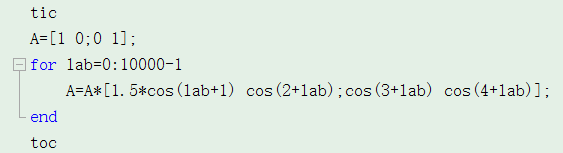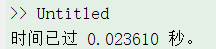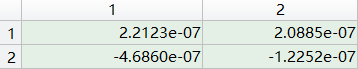用之前提到改进的做法：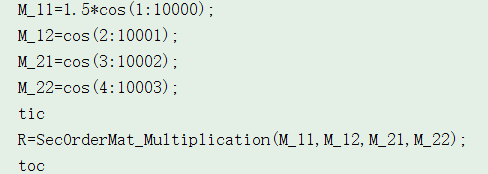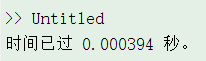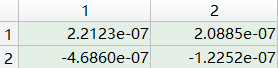快了10倍有木有……而且N越大效果越明显。实际上算光纤光栅，原本半小时的程序可以缩短到几十秒，还是蛮有用的。3. 代码%快速二阶矩阵连乘函数
%知乎 Floron
%--------------------------------------------------------------------------
function out=SecOrderMat_Multiplication(M_11,M_12,M_21,M_22)
mat_size=ceil((length(M_11))^0.5);
Length_full=mat_size^2;
M_11=[M_11 ones(1,Length_full-length(M_11))];
M_12=[M_12 zeros(1,Length_full-length(M_12))];
M_21=[M_21 zeros(1,Length_full-length(M_21))];
M_22=[M_22 ones(1,Length_full-length(M_22))];
M_11=reshape(M_11,mat_size,mat_size).';
M_12=reshape(M_12,mat_size,mat_size).';
M_21=reshape(M_21,mat_size,mat_size).';
M_22=reshape(M_22,mat_size,mat_size).';
row_M_11_0=1;
row_M_12_0=0;
row_M_21_0=0;
row_M_22_0=1;
for lab=1:mat_size
row_M_11=row_M_11_0.*M_11(:,lab)+row_M_12_0.*M_21(:,lab);
row_M_12=row_M_11_0.*M_12(:,lab)+row_M_12_0.*M_22(:,lab);
row_M_21=row_M_21_0.*M_11(:,lab)+row_M_22_0.*M_21(:,lab);
row_M_22=row_M_21_0.*M_12(:,lab)+row_M_22_0.*M_22(:,lab);
row_M_11_0=row_M_11;
row_M_12_0=row_M_12;
row_M_21_0=row_M_21;
row_M_22_0=row_M_22;
end
M_11=row_M_11';
M_12=row_M_12';
M_21=row_M_21';
M_22=row_M_22';
row_M_11_0=1;
row_M_12_0=0;
row_M_21_0=0;
row_M_22_0=1;
for lab=1:mat_size
row_M_11=row_M_11_0.*M_11(lab)+row_M_12_0.*M_21(lab);
row_M_12=row_M_11_0.*M_12(lab)+row_M_12_0.*M_22(lab);
row_M_21=row_M_21_0.*M_11(lab)+row_M_22_0.*M_21(lab);
row_M_22=row_M_21_0.*M_12(lab)+row_M_22_0.*M_22(lab);
row_M_11_0=row_M_11;
row_M_12_0=row_M_12;
row_M_21_0=row_M_21;
row_M_22_0=row_M_22;
end
out=[row_M_11,row_M_12;row_M_21,row_M_22];
end
%--------------------------------------------------------------------------
%                               介绍
%   当有许多二阶矩阵连乘：M_1*M_2*M_3*.....*M_n。（例如传输矩阵）
%   如果采用传统的for循环，计算次数为n次。
%   这里采用向量化的办法，对于连续相乘的矩阵，在不改变顺序的前提下可以随意添加
%括号，将其改写为一个sqrt(n)*sqrt(n)的方阵，沿着行增加的方向，所有列同步连乘，
%最终得到一列向量后再沿着列增加方向连乘。最终计算次数为2*sqrt(n)次。极大提升了
%计算速度，尤其是当n足够大时。
%--------------------------------------------------------------------------
%                               用法
%   将每个单独的矩阵（1,1）位置的元素作为一个行向量M_11输入。
%   将每个单独的矩阵（1,2）位置的元素作为一个行向量M_12输入。
%   将每个单独的矩阵（2,1）位置的元素作为一个行向量M_21输入。
%   将每个单独的矩阵（2,2）位置的元素作为一个行向量M_22输入。
%--------------------------------------------------------------------------
%                               示范
%   求[1,2;3,4]*[10,20;30,40]*[100,200;300,400]*[1000,2000;3000,4000]
%   输入分别为：
%   M_11=[1,10,100,1000]
%   M_12=[2,20,200,2000]
%   M_21=[3,30,300,3000]
%   M_22=[4,40,400,4000]
%--------------------------------------------------------------------------
%           代码示范（复制同一个文件夹的另一个m文件中使用）：
% % M_11=[1,2,3,4,5,6];
% % M_12=[2,3,4,5,6,7];
% % M_21=[3,4,5,6,7,8];
% % M_22=[4,5,6,7,8,9];
% % %use function
% % A_myfun=SecOrderMat_Multiplication(M_11,M_12,M_21,M_22);
% % %use for-loop
% % A=[1 0;0 1];
% % for lab=0:5
% %     A=A*[1+lab 2+lab;3+lab 4+lab];
% % end
%-------------------------------------------------------------------------- 如果有疑问可以留言，如果觉得好用请给我口米吃。展开全文• 前言今天来介绍一下MATLAB中corrcoef命令来计算相关系数矩阵，并且用imagesc和colorbar命令来画热力图。需要了解的预备知识1 单/双尾检测2 置信区间3 相关系数矩阵等等统计学基础知识参考资料MATLAB2016a官方文档，...前言今天来介绍一下MATLAB中corrcoef命令来计算相关系数矩阵，并且用imagesc和colorbar命令来画热力图。需要了解的预备知识1 单/双尾检测2 置信区间3 相关系数矩阵等等统计学基础知识参考资料MATLAB2016a官方文档，自己所学的知识，以及百度百科的相关介绍相关系数介绍corrcoef函数之前，我想介绍一下三种相关系数：1 Pearson（皮尔逊）相关系数2 Spearman（斯皮尔曼）相关系数3 kendall秩相关系数需要说明的是，在此函数中，用的均为皮尔逊相关系数，皮尔逊相关系数的具体适用范围请看这篇文章，已经介绍的十分详细了：伊豆：统计学习--三种常见的相关系数​zhuanlan.zhihu.comMATLAB中的corrcoef函数example1：R= corrcoef(A,B)returns coefficients between two random variablesAandB.即返回A与B（不限于A和B，可以有多个）的相关系数矩阵，即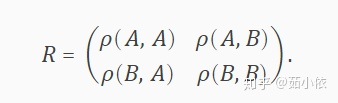由于自身与自身相关性为1，所以对角线元素均为1A = randn(10,1);
B = randn(10,1);
R = corrcoef(A,B)
R =

1.0000    0.4518
0.4518    1.0000example2：[R,P] = corrcoef(___)returns the matrix of correlation coefficients and the matrix of p-values for testing the hypothesis that there is no relationship between the observed phenomena (null hypothesis). Use this syntax with any of the arguments from the previous syntaxes. If an off-diagonal element ofPis smaller than the significance level (default is0.05), then the corresponding correlation inRis considered significant. This syntax is invalid ifRcontains complex elements.此时函数值可以是多列长度相同的向量，返回R矩阵和P矩阵，其中R矩阵与上面的含义相同，P矩阵是以某概率拒绝接受假设的概率（双尾检验），通俗来讲，认为当p小于0.05则可以认为这两个向量有显著相关性。x = randn(6,1);
y = randn(6,1);
z = randn(6,1);
A = [x y z];
[R,P] = corrcoef(A)
R =

1.0000    0.9087   -0.3804
0.9087    1.0000   -0.4119
-0.3804   -0.4119    1.0000

P =

1.0000    0.0121    0.4569
0.0121    1.0000    0.4170
0.4569    0.4170    1.0000example3：[R,P,RL,RU] = corrcoef(___)includes matrices containing lower and upper bounds for a 95% confidence interval for each coefficient. This syntax is invalid ifRcontains complex elements.返回值多了RL和RURL — Lower bound for correlation coefficientmatrixLower bound for correlation coefficient, returned as a matrix. RU — Upper bound for correlation coefficientmatrixUpper bound for correlation coefficient, returned as a matrix. 即RL是每个变量相关系数的下限，RU是每个变量相关系数的上限x = randn(6,1);
y = randn(6,1);
z = randn(6,1);
A = [x y z];
[R,P,RL,RU] = corrcoef(A)
R =

1.0000    0.4714   -0.5125
0.4714    1.0000   -0.5612
-0.5125   -0.5612    1.0000

P =

1.0000    0.3452    0.2985
0.3452    1.0000    0.2465
0.2985    0.2465    1.0000

RL =

1.0000   -0.5509   -0.9351
-0.5509    1.0000   -0.9432
-0.9351   -0.9432    1.0000

RU =

1.0000    0.9280    0.5120
0.9280    1.0000    0.4597
0.5120    0.4597    1.0000如何画热力图（即通过颜色的不同显示相关性的相对大小）A = [x1 x2 x3 x4 x5];
[R,P] = corrcoef(A);
imagesc(R);
colorbar效果：（可以在颜色编辑器更改自己的颜色偏好）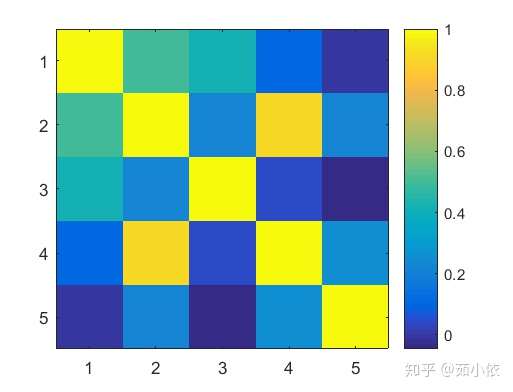专业词汇coefficient 系数variable 变量matrix 矩阵hypothesis 假设significance level 显著水平concordant 一致的discordant 不一致的
展开全文• PAGE / NUMPAGES matlab求解相关系数 最近收到一项新任务要求两个矩阵相关系数说白了就是转换成向量两两计算本 来这个工作我是想自己写个小程序搞定的但是大家纷纷反映matlab自带了此项功能本着活到老学到老的心态...
• %变量 para_all=[input_train_2017,input_train_2018,input_train_2019... ;output_train_2017,...%求相关系数矩阵 corr1=corrcoef(para_all'); %相关系数矩阵是对称的，令下三角矩阵保持不变，上三角置为0 corr...
• 最近收到一项新任务，要求两个矩阵相关系数，说白了就是转换成向量两两计算。本来这个工作我是想自己写个小程序搞定的，但是大家纷纷反映matlab自带了此项功能，本着活到老学到老的心态，我开始查找这个函数，目测...
• ## matlab绘制矩阵色块图

万次阅读 多人点赞 2018-06-09 20:12:48
在R语言中有根据矩阵绘制色块图的程序，可以用于绘制相关系数矩阵图，用丰富的颜色和形状形象的展示矩阵元素值的大小。今天介绍的是如何用matlab绘制矩阵色块图。 1、绘制网格线，网格中显示矩阵元素，显示黑色...
• ## Matlab求相关系数

千次阅读 2011-07-23 10:41:44
Matlab相关系数如何求一个矩阵中每一列与其他列的相关系数 A=rand(4,4);x=rand(4,1);for i=1:4;B=corrcoef(A(:,i),x);c(i)=B(1,2);end结果：c = 0.1438 0.7138 0.9718c
• 只是要一个相关数而已，cov，corrcoef函数为什么要出来矩阵呢，好麻烦，每次设置系数的时候都感觉不踏实。。然后就自己写了两个小函数，技术含量比较低，可以看作函数学习的例子吧。。然后默认系数都是1/N，需要改成...
• 摘要：MATLAB对于矩阵处理是非常高效的，而C++对于矩阵操作是非常麻烦的，因而可以采用C++与MATLAB混合编程求解矩阵问题。主要思路就是，在MATLAB中编写函数脚本并使用C++编译为dll文件(在C++中可以调用编译的函数)...
• 一、matlab相关系数计算例：corr1=corrcoef(x,y),结果即为包含x，y相关系数的一个2×2矩阵，主对角线为自相关系数1，副对角线为x与y的线性相关系数用的是pearson相关。corr2=corrcoef(x,y),结果为x与y的线性相关...
• 通过与老师商议讨论重新制定了原始数据表，而在筛选过程中，有一个步骤是独立性检验，我们通过计算机来判断是否通过独立性检验，其中包括计算数据矩阵相关系数和显著性。重新制定后的表如下图，我们这里用Excel表...
• 版权声明：本文为博主原创文章，遵循 CC 4.0 BY-SA 版权协议，转载请附上原文出处链接和本声明。... 在R语言中有根据矩阵绘制色块图的程序，可以用于绘制相关系数矩阵图，用丰富的颜色和形状形象的...
• ## 利用MATLAB绘制矩阵色块图

万次阅读 多人点赞 2019-06-18 21:11:35
R语言中有一个根据实值矩阵绘制色块图的程序（用于绘制相关系数矩阵图），可以用丰富的颜色和形状形象的展示矩阵元素值的大小。遗憾的是MATLAB中没有这样的函数，因此我就用MATLAB编写了一个matrixplot函数，下面给...
• R = corrcoef(A) 返回 A 的相关系数矩阵，其中 A 的列表示随机变量，行表示观测值。 示例 R = corrcoef(A,B) 返回两个随机变量 A 和 B 之间的系数。 示例 [R,P] = corrcoef(___) 返回相关系数
• 在机器学习模式识别相关算法中，经常需要求样本的协方差矩阵C和散布矩阵S。如在PCA主成分分析中，就需要计算样本的散度矩阵，而有的教材资料是计算协方差矩阵。实质上协方差矩阵和散度矩阵的意义就是一样的，散布...
• matlab里如何产生方差均值已知的服从正态分布的随机数？ matlab中已知协方差矩阵怎www.zhiqu.org 时间： 2020-12-14mvnrnd(mu,...1 4],100)已知协方差矩阵，计算相关系数可以按图中的公式进行。R就是相关系数矩...
• 这是求相关度的结果，对于一般的矩阵X，执行A=corrcoef(X)后，A中每个值的所在行a和列b，反应的是原矩阵X中相应的第a个列向量和第b个列向量的相似程度(即相关系数)。计算公式是：C(1,2)/SQRT(C(1,1)*C(2,2))，其中C...
• 相关系数函数代码函数调用 1.均值 求出图像矩阵所有数值的均值。 注意点： ①图像矩阵的每个值都是uint8类型的，uint8的范围是0-255，在进行求和之前需要把图像矩阵转换成double类型，如果不转换，继续对uint8类型...图像处理
• 对于一般的矩阵X，执行A=corrcoef(X)后，A中每个值的所在行a和列b，反应的是原矩阵X中相应的第a个列向量和第b个列向量的相似程度(即相关系数)。计算公式是：C(1,2)/SQRT(C(1,1)*C(2,2))，其中C表示矩阵[f,g]的协方差...
• 简单来说：皮尔逊相关系数描述线性相关性的强弱 皮尔逊相关系数： 皮尔逊相关系数：即消除...MATLAB计算皮尔逊相关系数： clear;clc; load 'data.mat' R = corrcoef(data) % correlation coefficient 判定相关性解.数学建模
• 二、总体皮尔逊Person相关系数 三、样本皮尔逊Person相关系数 四、相关性可视化 五、关于皮尔逊相关系数的一些理解误区 （一）容易犯错的点 （二）总结 六、对相关系数大小的解释 七、例题——计算皮尔逊相关...
• mean（X）:对矩阵A求列平均；mean（X,2）对矩阵X求行平均；若X为向量，mean（X）返回X中平均。 分母为n时，是整体方差 方差:Y=var(X,0,1):求矩阵X的列向量方差； Y=var(X,0,2):求矩阵X的行向量方差 协方差......

# matlab相关系数矩阵matlab 订阅